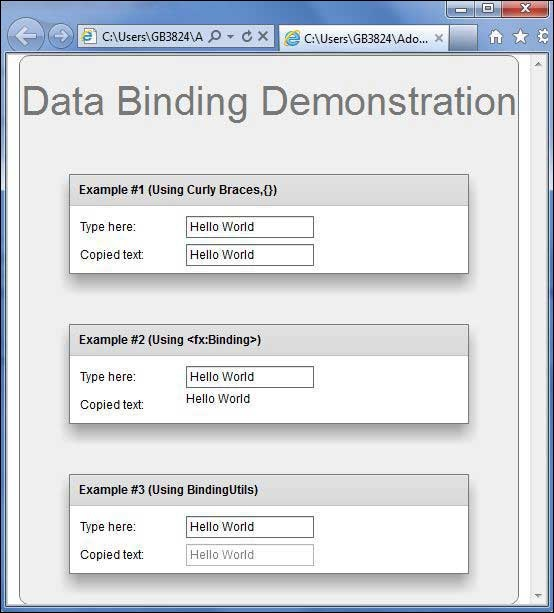# Flex - Data Binding

## What is Data Binding?

Data Binding is a process in which data of one object is tied to another object. It requires a source property, a destination property and a triggering event which indicates, when to copy the data from source to destination.

Flex provides three ways to do Data Binding as below

• Curly brace syntax in MXML Script ({})
• <fx:binding> tag in MXML
• BindingUtils in ActionScript

### Data Binding – Using Curly Braces in MXML

The following example demonstrates how to use curly braces to specify data binding of a source to destination.

```<s:TextInput id = "txtInput1" />
<s:TextInput id = "txtInput2" text = "{txtInput1.text}" />
```

### Data Binding – Using <fx:Binding> tag in MXML

The following example demonstrates how to use tag to specify data binding of a source to destination.

```<fx:Binding source = "txtInput1.text" destination = "txtInput2.text" />
<s:TextInput id = "txtInput1" />
<s:TextInput id = "txtInput2" />
```

### Data Binding – Using BindingUtils in ActionScript

The following example demonstrates how to use BindingUtils to specify data binding of a source to destination.

```<fx:Script>
<![CDATA[
import mx.binding.utils.BindingUtils;
import mx.events.FlexEvent;

protected function txtInput2_preinitializeHandler(event:FlexEvent):void {
BindingUtils.bindProperty(txtInput2,"text",txtInput1, "text");
}
]]>
</fx:Script>

<s:TextInput id = "txtInput1" />
<s:TextInput id = "txtInput2"
preinitialize = "txtInput2_preinitializeHandler(event)" />
```

## Flex Data Binding Example

Let us follow the steps given below to see skinning in action in a Flex application by creating a test application −

Step Description
1 Create a project with a name HelloWorld under a packagecom.tutorialspoint.client as explained in the Flex - Create Application chapter.
2 Modify HelloWorld.mxml as explained below. Keep rest of the files unchanged.
3 Compile and run the application to make sure business logic is working as per the requirements.

Following is the content of the modified HelloWorld.mxml filesrc/com/tutorialspoint/client/HelloWorld.mxml.

```<?xml version = "1.0" encoding = "utf-8"?>
width = "100%" height = "100%" minWidth = "500" minHeight = "500">

<fx:Style source = "/com/tutorialspoint/client/Style.css" />
<fx:Script>
<![CDATA[
import mx.binding.utils.BindingUtils;
import mx.events.FlexEvent;

protected function txtInput6_preinitializeHandler(event:FlexEvent):void {
BindingUtils.bindProperty(txtInput6,"text",txtInput5, "text");
}
]]>
</fx:Script>

<fx:Binding source = "txtInput3.text" destination = "txtInput4.text" />
<s:BorderContainer width = "500" height = "550" id = "mainContainer"
styleName = "container">
<s:VGroup width = "100%" height = "100%" gap = "50" horizontalAlign = "center"
verticalAlign = "middle">
<s:Label id = "lblHeader" text = "Data Binding Demonstration"
fontSize = "40" color = "0x777777" styleName = "heading" />
<s:Panel title = "Example #1 (Using Curly Braces,\{\})" width = "400"
height = "100" >
<s:layout>
</s:layout>

<s:HGroup >
<s:Label text = "Type here: " width = "100" paddingTop = "6" />
<s:TextInput id = "txtInput1" />
</s:HGroup>

<s:HGroup >
<s:Label text = "Copied text: " width = "100" paddingTop = "6" />
<s:TextInput id = "txtInput2" text = "{txtInput1.text}" />
</s:HGroup>
</s:Panel>

<s:Panel title = "Example #2 (Using &lt;fx:Binding&gt;)" width = "400"
height = "100" >
<s:layout>
</s:layout>

<s:HGroup >
<s:Label text = "Type here: " width = "100" paddingTop = "6" />
<s:TextInput id = "txtInput3" />
</s:HGroup>

<s:HGroup >
<s:Label text = "Copied text: " width = "100" paddingTop = "6" />
<s:Label id = "txtInput4" />
</s:HGroup>
</s:Panel>

<s:Panel title = "Example #3 (Using BindingUtils)" width = "400"
height = "100" >
<s:layout>
</s:layout>

<s:HGroup >
<s:Label text = "Type here: " width = "100" paddingTop = "6" />
<s:TextInput id = "txtInput5" />
</s:HGroup>

<s:HGroup >
<s:Label text = "Copied text: " width = "100" paddingTop = "6" />
<s:TextInput enabled = "false" id = "txtInput6"
preinitialize = "txtInput6_preinitializeHandler(event)" />
</s:HGroup>
</s:Panel>
</s:VGroup>
</s:BorderContainer>
</s:Application>
```

Once you are ready with all the changes done, let us compile and run the application in normal mode as we did in Flex - Create Application chapter. If everything is fine with your application, it will produce the following result: [ Try it online ]